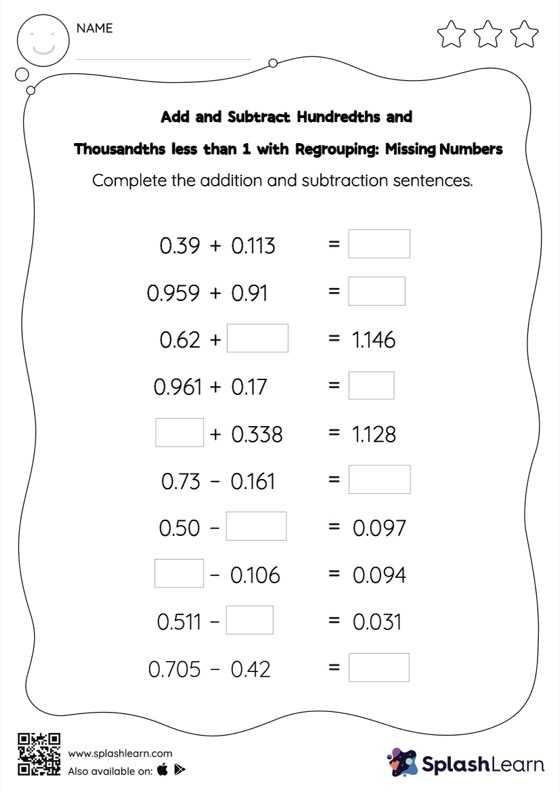# Add and Subtract Hundredths and Thousandths less than 1 with Regrouping: Missing Numbers Worksheet

Home > Add and Subtract Hundredths and Thousandths less than 1 with Regrouping: Missing NumbersWhile adding and subtracting decimals, students use the relationship between addition and subtraction to find the missing number. They also need to regroup numbers in add and subtract hundredths and thousandths less than 1 with regrouping worksheet utilizing the relationship between ones, tenth, hundredth, thousandth, etc., To get to the result. This worksheet is about practicing with the horizontal format in which numbers are written side by side. To develop flexibility with numbers and operations, students need to have enough practice in this format and not just rely on the vertical/column method.